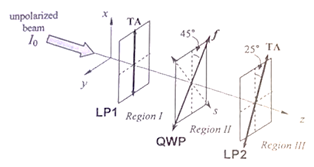# Problem: A beam of polarized light of intensity I 0 travels in the +z-direction and is incident on two linear polarizers (LP1 and LP2) and a quarter wave plate (QWP) as shown below, LP1 has a transmission axes (TA) parallel to the x-axis, the QWP has a fast axis oriented 45° with respect to the x-axis and LP2 has a TA oriented 25° with respect to the x-axis. The light in Regions I, II, and III has intensities I1, I2 and I3, respectively. Find I3/I2. a, I3/I2 = 0.82 b. I3/I2 = 0.18 c. I3/I2 = 1.0 d. I3/I2 = 0.88 e. I3/I2 = 0.50

###### FREE Expert Solution
80% (366 ratings)
###### Problem Details

A beam of polarized light of intensity I 0 travels in the +z-direction and is incident on two linear polarizers (LP1 and LP2) and a quarter wave plate (QWP) as shown below, LP1 has a transmission axes (TA) parallel to the x-axis, the QWP has a fast axis oriented 45° with respect to the x-axis and LP2 has a TA oriented 25° with respect to the x-axis. The light in Regions I, II, and III has intensities I1, I2 and I3, respectively. Find I3/I2.

a, I3/I2 = 0.82

b. I3/I2 = 0.18

c. I3/I2 = 1.0

d. I3/I2 = 0.88

e. I3/I2 = 0.50